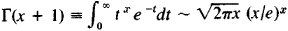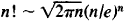Asymptotic Expression

The following article is from The Great Soviet Encyclopedia (1979). It might be outdated or ideologically biased.

Asymptotic Expression

a comparatively simple elementary function, approximately equal (with as small a relative error as desired) to a more complex function at large values of the argument (or for argument values that are close to a given value—for example, zero). An asymptotic expression is sometimes called an asymptotic formula or estimate. The exact definition is the following: A function φ (x) is an asymptotic expression for f (x) as x → ∞ (or xa), if f (x) / φ (x) → 1 as x (or xa), or, to put it differently, if f (x) = φ(x) [l + a (x)], where a (x) → 0 as x (or xa). In this case it is written: f (x) ~ φ (x) as xφ (or xa). As a rule, φ (x) must be an easily computable function. The simplest examples of asymptotic expressions for x → 0 are sin xx, tan xx, cot x ∼ 1/x, 1 − cos xx2/2, ln (1 + x) ∼ x, ax − 1 ∼ x In a (a > 0, a ≠ 1). More complex asymptotic expressions as x arise for important functions from the theory of numbers and special functions of mathematical physics. For example, π (x) ∼ x/ln x, where π (x) is the number of prime numbers not exceeding x:where ┌ (u) is the gamma function; for integer-number values x = n we have ┌(n + 1) = n!, which reduces to Stirling’s formula:as n. Still more complex asymptotic expressions are found, for example in Bessel functions.

Asymptotic expressions are also considered in the complex plane, z = x + iy. Thus, for example, ǀsin(x + iy)ǀ ∼eǀvǀ2 as yφ and yφ.

An asymptotic expression is, in general, a particular case (principal term) of more complex (and accurate) approximate expressions, which are called asymptotic series or expansions.

LITERATURE

Debruijn, N. G.Asimptoticheskie methody v analize. Moscow, 1961. (Translated from English.)

Evgrafov, M. A. Asymptoticheskie otsenki i tselye funktsii, 2nd ed. Moscow, 1962.

V. I. LEVIN

References in periodicals archive ?
We further pursue the asymptotic analysis for the ergodic sum rate and derive the asymptotic expression for the ergodic sum rate of NOMA relaying network over Nakagami-m fading channels.
The square modulus of asymptotic expression (13) approximates the survival probability over long times:
The asymptotic expression for the CDF of [[gamma].sup.F,ins.sub.R] at the high SNR when I is proportional to P is given as
Since both parts contributing to the win probability have the same factor [u.sub.1], it now suffices to maximize the sum [F.sub.1]([j.sub.2]) + [F.sub.2]([u.sub.1], [j.sub.2]), conditioning on [u.sub.1], that is we must maximize the asymptotic expression
For the general renewal risk model, an asymptotic expression For the finite time ruin probability is presented in ; that is,
(ii) We derive the asymptotic expression for outage probability of the system with feedback delay and CCI based on the closed-form expression of outage probability.
In order to provide insight into the impact of the different system parameters on performance, a simple and compact asymptotic expression of the BER for the high SNR regime is also derived and it is shown that correlation results in an increased BER for high values of the SNR.
Therefore, combining (67), (68), and (72), we derive an asymptotic expression for the protection seller, which is given by
*sin(pi* (1:N+1)'/(N+2)); a = idst(fx); % coefficients for Fejer's second rule % Filippi points % f evaluated at these points % Chebyshev coefficients If N > 2000, [Q.sub.N+1] ([alpha], [beta]) is computed by using the asymptotic expression (20) with the first four-term truncation.
With these parameter values, and using the asymptotic expression for the t-statistic (not shown), it is easy to calculate that, as [EXPRESION MATEMATICA IRREPRODUCIBLE EN ASCII].
Similarly from Equation (46) we have the next asymptotic expression for the electric field
J.M.Alonso and R.Qrtega in  have studied the dynamics of a class of mappings defined on the plane, which have an asymptotic expression

Site: Follow: Share:
Open / Close